Example 102.3 Analysis without Surface Trend Removal

This example uses PROC VARIOGRAM without removing potential surface trends in a data set in order to investigate a distinguished spatial direction in the data. In doing so, this example also serves as a guide to examine under which circumstances you might be able to bypass the effect of a trend on a semivariogram. Typically though, for theoretical semivariogram estimations you follow the analysis presented in An Anisotropic Case Study with Surface Trend in the Data.

As explained in the section Details: VARIOGRAM Procedure, when you compute the empirical semivariance for data that contain underlying surface trends, the outcome is the pseudo-semivariance. Pseudo-semivariograms are not estimates of the theoretical semivariogram; hence, they provide no information about the spatial continuity of your SRF.

However, in the section Empirical Semivariograms and Surface Trends it is mentioned that you might still be able to perform a semivariogram analysis with potentially non-trend-free data, if you suspect that your measurements might be trend-free across one or more specific directions. The example demonstrates this approach.

Reconsider the ozone data presented at the beginning of An Anisotropic Case Study with Surface Trend in the Data. The spatial distribution of the data is shown in Output 102.2.1, and the pairwise distance distribution for NHCLASSES=35 is illustrated in Output 102.2.3. This exploratory analysis suggested a LAGDISTANCE=4 km, and Output 102.2.2 indicated that for this LAGDISTANCE= you can consider a value of MAXLAGS=16.

Recall from the section Empirical Semivariograms and Surface Trends that you need to investigate the empirical semivariogram of the data in a few different directions in order to identify a trend-free direction. If such a direction exists, then you can proceed with this special type of analysis. The following statements employ NDIRECTIONS=8 to examine eight directions:

ods graphics on;
proc variogram data=ozoneSet plot(only)=semivar;
compute lagd=4 maxlag=16 ndirections=8 robust;
coord xc=East yc=North;
var Ozone;
run;

By default, the range of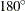is divided into eight equally distanced angles: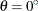,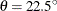,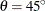,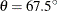,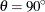,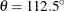,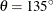, and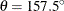. The resulting empirical semivariograms for these angles are shown in Output 102.3.1.

Output 102.3.1: Ozone Empirical Semivariograms with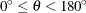and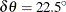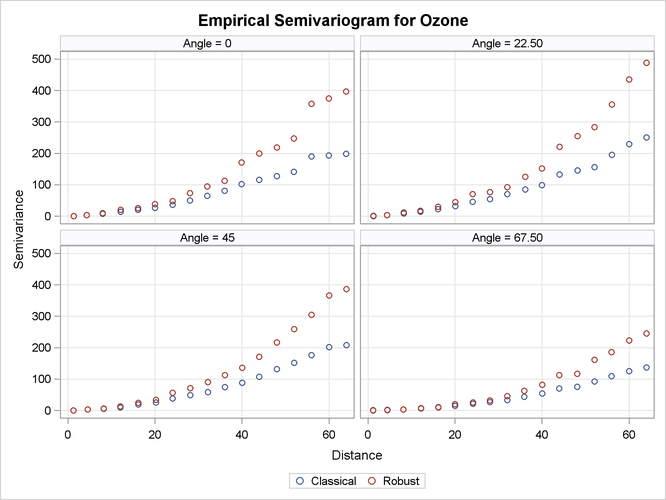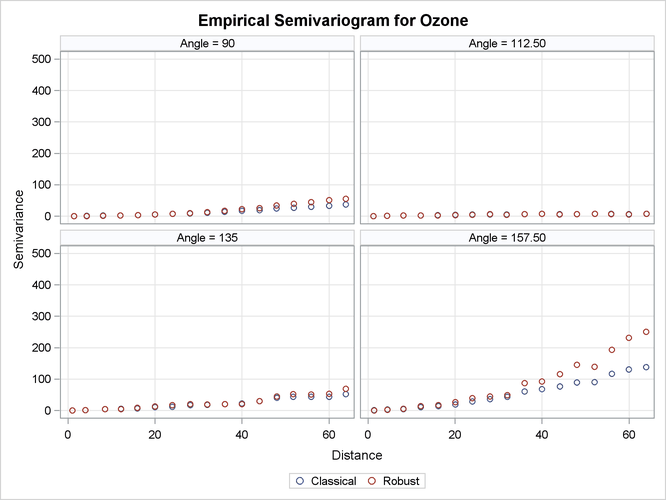The figures in Output 102.3.1 suggest an overall continuing increase with distance of the semivariance in all directions. As explained in the section Theoretical Semivariogram Models, this can be an indication of systematic trends in the data. However, the direction ofclearly indicates that the increase rate, if any, is smaller than the corresponding rates across the rest of the directions. You then want to search whether there exists a trend-free direction in the neighborhood of this angle.

Run PROC VARIOGRAM again, specifying several directions within an interval of angles where you want to close in and you suspect the existence of a trend-free direction. In the following step you specify ANGLETOL=15, which is smaller than the default value of 22.5, and you also specify BANDWIDTH=10 km. The smaller values help with minimization of the interference with neighboring directions, as discussed in the section Angle Classification.

The aforementioned considerations are addressed in the following statements:

proc variogram data=ozoneSet plot(only)=semivar(cla);
compute lagd=4 maxlag=16 robust;
directions 100(15,10) 103(15,10)
106(15,10) 108(15,10)
110(15,10) 112(15,10)
115(15,10) 118(15,10);
coord xc=East yc=North;
var Ozone;
run;

Your analysis has brought you to examine a narrow strip of angles within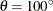and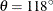. The pseudo-semivariograms in Output 102.3.2 and Output 102.3.3 indicate that at the boundaries of this strip, the angles display increasing semivariance with distance. On the other hand, within this interval there are directions across which the semivariance is tentatively reaching a sill, and these are potential candidates to be trend-free directions.

Output 102.3.2: Ozone Empirical Semivariograms in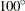,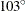,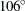, and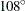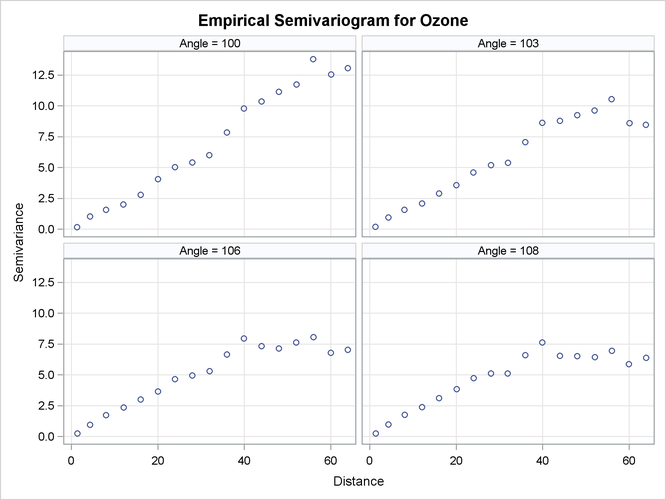Output 102.3.3: Ozone Empirical Semivariograms in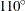,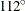,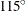, and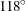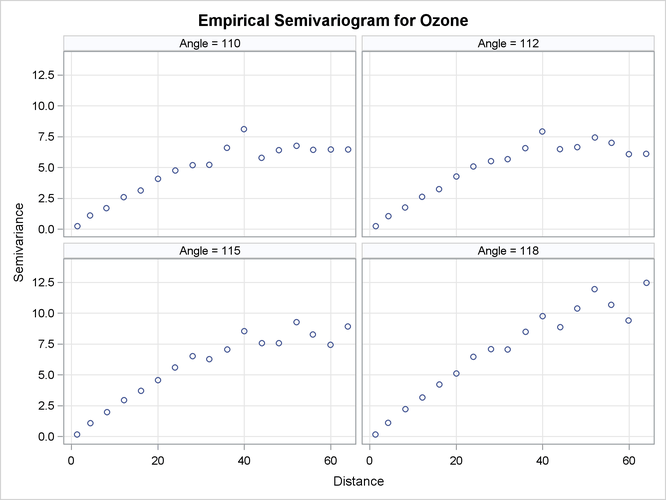You can further investigate this angle spectrum in more detail. For example, you can monitor additional angles in between, or use a smaller LAGDISTANCE= and increased MAXLAGS= values to single out the most qualified candidate. For the purpose of this example, you can consider the direction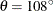to very likely be the trend-free one you are looking for.

From a physical standpoint, the trend-free direction, if it exists, is expected to be perpendicular to the direction of the maximum dip in the values of the ozone field, as mentioned in the section Empirical Semivariograms and Surface Trends. If you cross-examine the ozone data distribution in Output 102.2.1, the figure suggests that this direction exists and is slightly tilted clockwise with respect to the E–W axis. This direction emerges from the mild stratification of the ozone values in your data distribution. The ozone concentrations across it are similar when compared to surrounding directions, and as such, it has been identified as a trend-free direction.

Your next step is to obtain the empirical semivariogram in the suspected trend-free direction ofand to perform a theoretical model fit.

The semivariance in Output 102.3.2 exhibits a slow, almost linear rise at short distances and seems to be reaching the sill fast, rather than asymptotically. You can accommodate this behavior by using the spherical model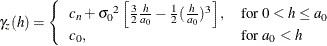where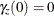and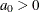. The empirical semivariograms also suggest that there does not seem to be a nugget effect. Assume that in this example you are interested in what the fitting process concludes about the nugget effect, so you skip the NUGGET= option in the MODEL statement. You also let PROC VARIOGRAM provide initial values for the rest of the model parameters. Eventually, you use the PLOTS option to inspect the classical and robust empirical semivariograms in the selected direction and to produce a plot of the fitted model. The following statements implement these considerations:

proc variogram data=ozoneSet plot(only)=(semivar fit);
compute lagd=4 maxlag=16 robust cl;
directions 108(15,10);
coord xc=East yc=North;
model form=sph;
var ozone;
run;

ods graphics off;

The classical and robust empirical semivariograms in the selected directionare displayed in Output 102.3.4.

Output 102.3.4: Ozone Classical and Robust Empirical Semivariograms in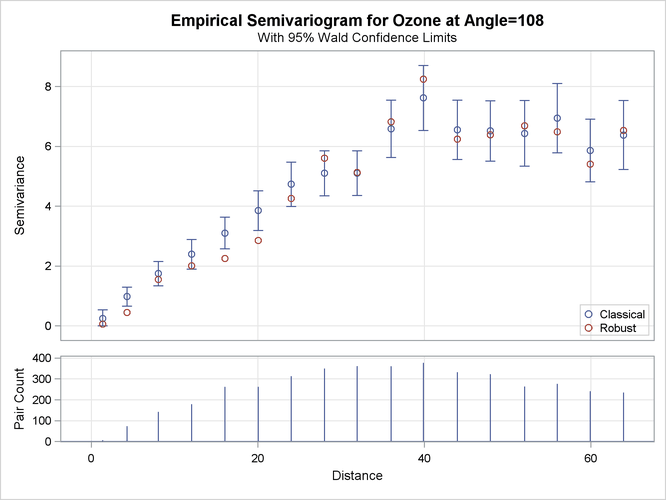The output continues with information about the fitting process, which terminates successfully and produces the estimated parameters and the fit summary tables shown in Output 102.3.5. The near-zero nugget parameter estimate indicates that you can consider the process to be practically free of nugget effect.

Output 102.3.5: Weighted Least Squares Fitting Parameter Estimates and Summary inParameter Estimates
Parameter Estimate Approx
Std Error
DF t Value Approx
Pr > |t|
Nugget 0.006260 0.09449 14 0.07 0.9481
Scale 6.6791 0.1741 14 38.37 <.0001
Range 47.3012 2.0776 14 22.77 <.0001

Fit Summary
Model Weighted
SSE
AIC
Sph 13.13869 1.61991

The fitted and empirical semivariograms for the selected directionare displayed in Output 102.3.6.

Output 102.3.6: Fitted Theoretical and Empirical Semivariogram for the Ozone Data in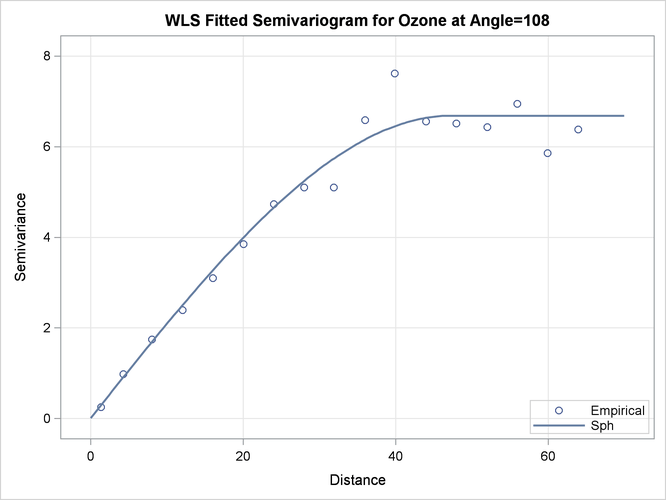A comparative look at the empirical and fitted semivariograms in Output 102.3.6 and Output 102.2.12 suggests that the analysis of the trend-free ResidualOzone produces a different outcome from that of the original Ozone values. In fact, a more suitable comparison can be made between the semivariograms in the assumed trend-free directionof the current scenario and the one shown in Output 102.2.6 in the nearly identical direction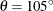. It might seem unreasonable that these two semivariograms are produced both in the same ozone study and in a narrow band of directions free of apparent surface trends, yet they bear no resemblance. However, the lack of similarity in these plots stems from operating on two different data sets where the outcome depends on the actual data values.

More specifically, the semivariogram analysis treats the trend-free ozone set and the original ozone measurements as different quantities. The process of detrending the original Ozone values is a transformation of these values into the trend-free values of ResidualOzone. Any existing spatial correlation in the original data is not necessarily retained within the transformed data. Depending on the transformation features, the emerging data set has its own characteristics, as demonstrated in this example.

A final remark concerns the issue of isotropy. Based on the details presented in the section Empirical Semivariograms and Surface Trends, your knowledge of the spatial structure of the ozoneSet data set is limited to the selected trend-free direction you indicated in the present example. You can generalize this outcome for all spatial directions only if you consider the hypothesis of isotropy in the ozone field to be reasonable. However, you cannot infer the assumption of anisotropy in the present example based on the analysis in the section Analysis with Surface Trend Removal. Again, the reason is that you currently use the observed Ozone values, whereas the ResidualOzone data in the previous example emerged from a transformation of the current data. Hence, you have essentially two data sets that do not necessarily share the same properties.